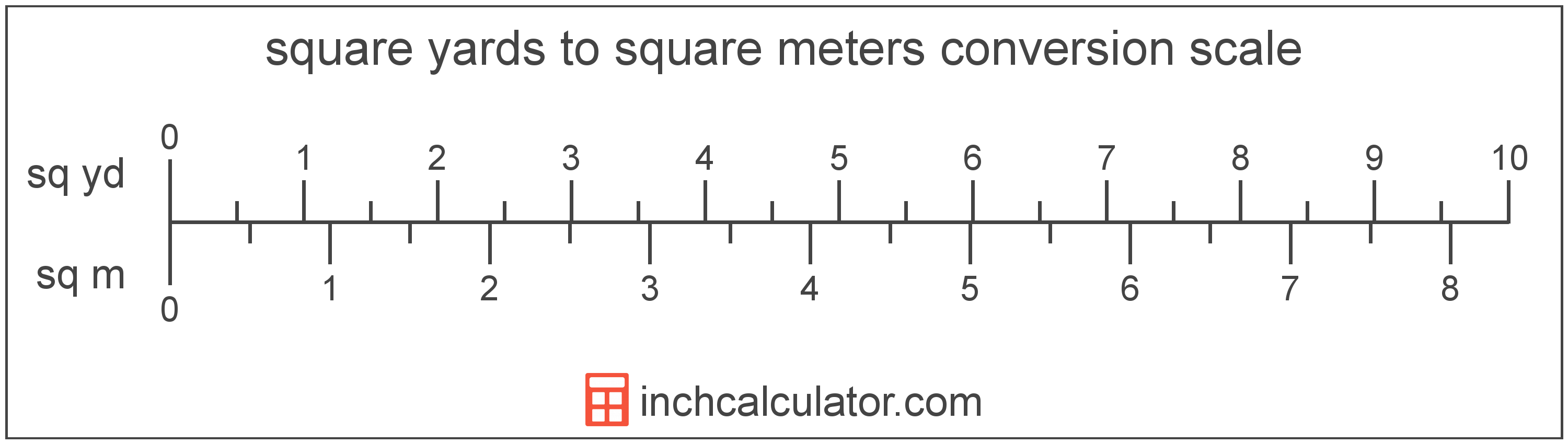# Square Yards to Square Meters Converter

Enter the area in square yards below to get the value converted to square meters.

(find square yards)
Results in Square Meters:1 sq yd = 0.836127 sq m

Do you want to convert square meters to square yards?

## How to Convert Square Yards to Square Meters

To convert a measurement in square yards to a measurement in square meters, multiply the area by the following conversion ratio: 0.836127 square meters/square yard.

Since one square yard is equal to 0.836127 square meters, you can use this simple formula to convert:

square meters = square yards × 0.836127

The area in square meters is equal to the area in square yards multiplied by 0.836127.

For example, here's how to convert 5 square yards to square meters using the formula above.
square meters = (5 sq yd × 0.836127) = 4.180637 sq m### How Many Square Meters Are in a Square Yard?

There are 0.836127 square meters in a square yard, which is why we use this value in the formula above.

1 sq yd = 0.836127 sq m

## What Is a Square Yard?

One square yard is equivalent to the area of a square with sides that are each 1 yard in length. One square yard is roughly equal to 9 square feet or 0.836127 square meters.

The square yard is a US customary and imperial unit of area. A square yard is sometimes also referred to as a square yd. Square yards can be abbreviated as sq yd, and are also sometimes abbreviated as yd². For example, 1 square yard can be written as 1 sq yd or 1 yd².

You can use a square yards calculator to calculate the area of a space if you know its dimensions.

## What Is a Square Meter?

One square meter is equivalent to the area of a square with sides that are each 1 meter in length. One square meter is equal to 10.76391 square feet or 10,000 square centimeters.

The square meter, or square metre, is the SI derived unit for area in the metric system. A square meter is sometimes also referred to as a square m. Square meters can be abbreviated as sq m, and are also sometimes abbreviated as . For example, 1 square meter can be written as 1 sq m or 1 m².

You can use a square meters calculator to calculate the area of a space if you know its dimensions.

## Square Yard to Square Meter Conversion Table

Table showing various square yard measurements converted to square meters.
Square Yards Square Meters
1 sq yd 0.836127 sq m
2 sq yd 1.6723 sq m
3 sq yd 2.5084 sq m
4 sq yd 3.3445 sq m
5 sq yd 4.1806 sq m
6 sq yd 5.0168 sq m
7 sq yd 5.8529 sq m
8 sq yd 6.689 sq m
9 sq yd 7.5251 sq m
10 sq yd 8.3613 sq m
11 sq yd 9.1974 sq m
12 sq yd 10.03 sq m
13 sq yd 10.87 sq m
14 sq yd 11.71 sq m
15 sq yd 12.54 sq m
16 sq yd 13.38 sq m
17 sq yd 14.21 sq m
18 sq yd 15.05 sq m
19 sq yd 15.89 sq m
20 sq yd 16.72 sq m
21 sq yd 17.56 sq m
22 sq yd 18.39 sq m
23 sq yd 19.23 sq m
24 sq yd 20.07 sq m
25 sq yd 20.9 sq m
26 sq yd 21.74 sq m
27 sq yd 22.58 sq m
28 sq yd 23.41 sq m
29 sq yd 24.25 sq m
30 sq yd 25.08 sq m
31 sq yd 25.92 sq m
32 sq yd 26.76 sq m
33 sq yd 27.59 sq m
34 sq yd 28.43 sq m
35 sq yd 29.26 sq m
36 sq yd 30.1 sq m
37 sq yd 30.94 sq m
38 sq yd 31.77 sq m
39 sq yd 32.61 sq m
40 sq yd 33.45 sq m

## References

1. Collins Dictionary, Definition of 'square meter', https://www.collinsdictionary.com/us/dictionary/english/square-meter